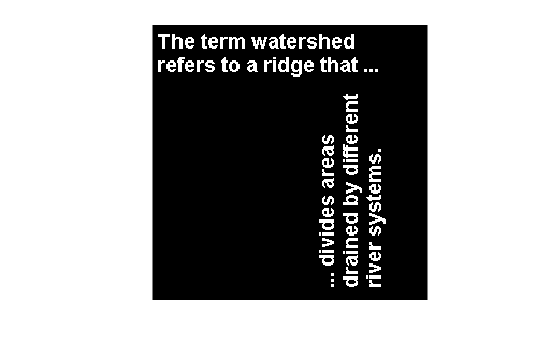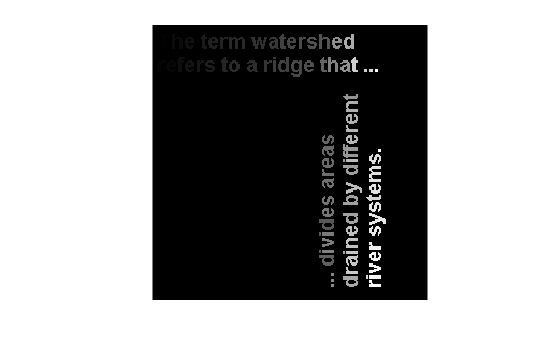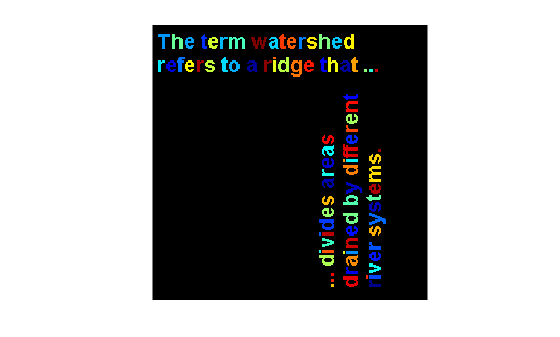# labelmatrix

Create label matrix from `bwconncomp` structure

## Syntax

``L = labelmatrix(CC)``

## Description

A label matrix labels objects or connected components in a binary image with unique integer values. Use a label matrix to visualize distinct objects or connected components.

example

````L = labelmatrix(CC)` creates a label matrix, `L`, from the connected components structure `CC` returned by `bwconncomp`. ```

## Examples

collapse all

Read a binary image into the workspace. Display the image.

```BW = imread('text.png'); imshow(BW)```Calculate the connected components using `bwconncomp`.

`CC = bwconncomp(BW);`

Create a label matrix using `labelmatrix`. Each label has a unique numeric index.

`L = labelmatrix(CC);`

Find the maximum value of the label matrix. This value indicates the number of detected objects, in this case, 88.

`numObjects = max(L(:))`
```numObjects = uint8 88 ```

Display the label matrix as an image. Because the maximum label value is much smaller than the maximum value of the `uint8` data type, increase the display range of the image to make the labels more distinct.

`imshow(L,[])`It is challenging to see the objects labeled with small label values. Further, it is challenging to differentiate objects with comparable label values. To make it easier to differentiate the different connected components, display the label matrix as an RGB image using `label2rgb` and shuffle the color order of the labels.

`imshow(label2rgb(L,'jet','k','shuffle'));`## Input Arguments

collapse all

Connected components, specified as a structure with four fields.

FieldDescription
`Connectivity`Connectivity of the connected components (objects)
`ImageSize`Size of the binary image
`NumObjects`Number of connected components (objects) in the binary image.
`PixelIdxList`1-by-`NumObjects` cell array where the k-th element in the cell array is a vector containing the linear indices of the pixels in the k-th object.

## Output Arguments

collapse all

Label matrix of contiguous regions, returned as matrix of nonnegative integers. The pixels labeled `0` are the background. The pixels labeled `1` make up one object; the pixels labeled `2` make up a second object; and so on.

The size of `L` is determined by the value of the `CC.ImageSize` field. The class of `L` depends upon the number of contiguous regions. `labelmatrix` uses the smallest class that can represent the number of objects, `CC.NumObjects`, as shown in the table.

ClassRange
`'uint8'`

`CC.NumObjects` $\le$ 255

`'uint16'`

256 $\le$ `CC.NumObjects` $\le$ 65535

`'uint32'`

65536 $\le$ `CC.NumObjects` $\le {2}^{32}-1$

`'double'`

`CC.NumObjects` $\ge {2}^{32}$

Data Types: `double` | `uint8` | `uint16` | `uint32`

## Version History

Introduced in R2009a